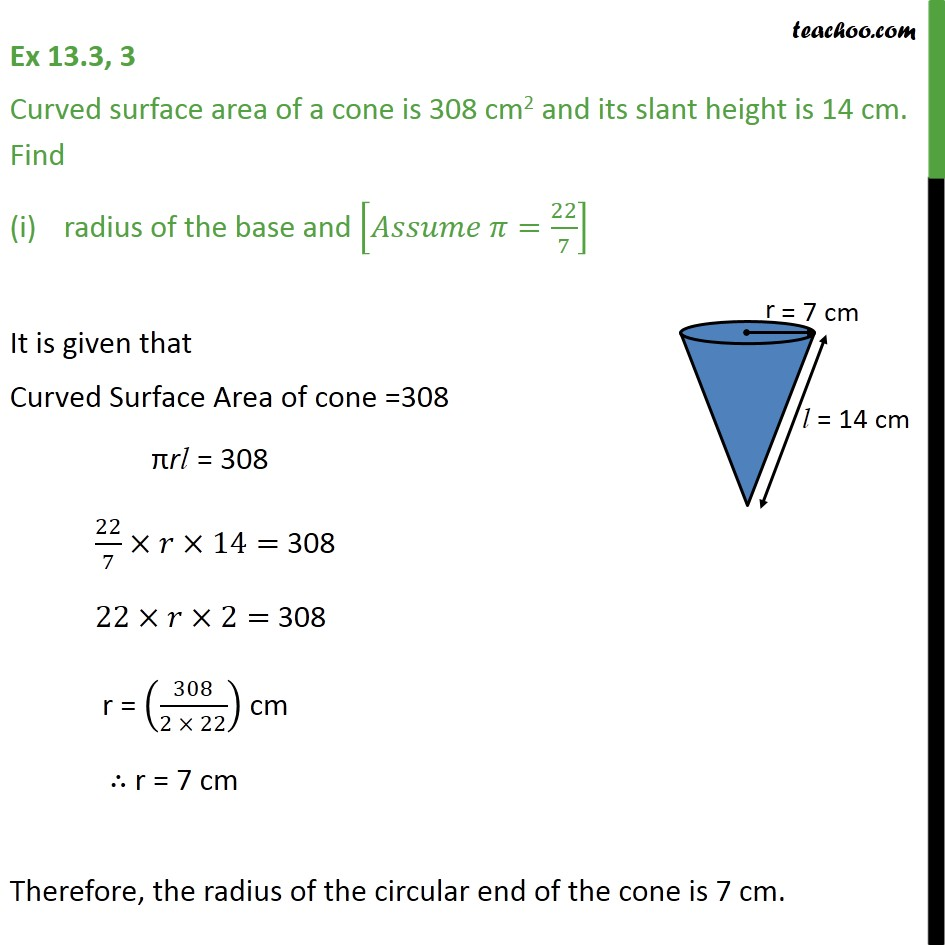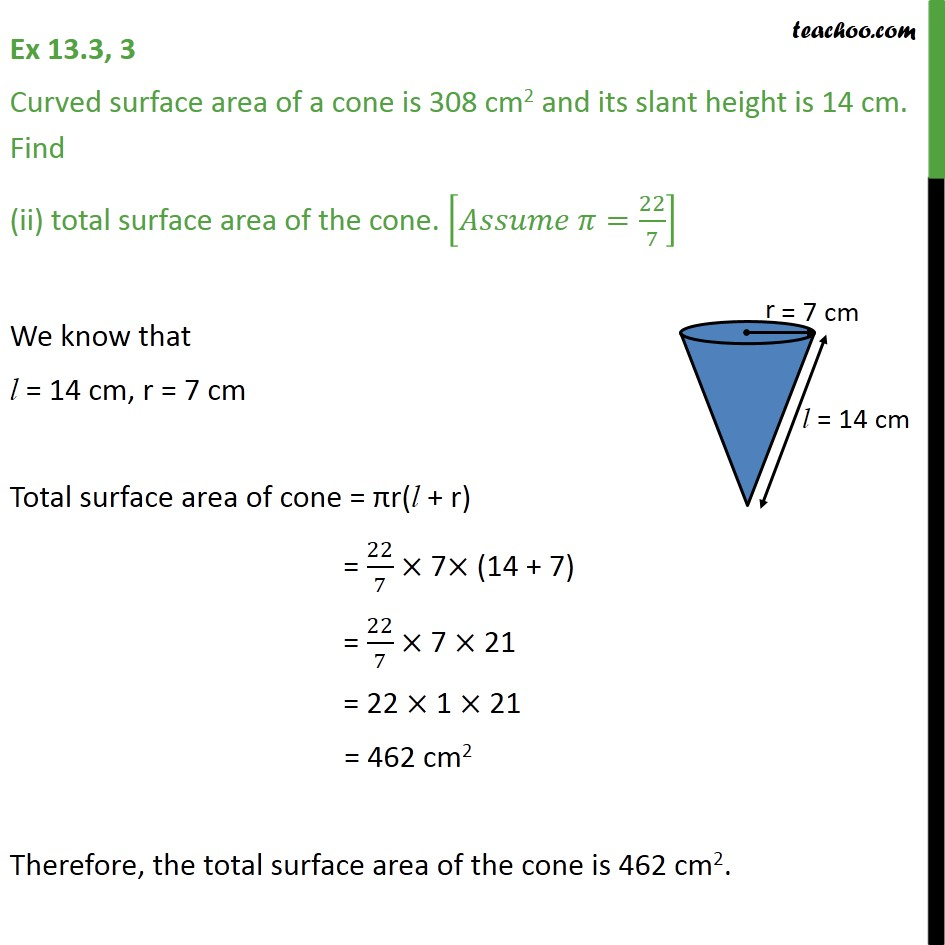Chapter 13 Class 9 Surface Areas and Volumes

Class 9
Important Questions for Exam - Class 9Learn in your speed, with individual attention - Teachoo Maths 1-on-1 Class

### Transcript

Ex 11.1, 3 Curved surface area of a cone is 308 cm2 and its slant height is 14 cm. Find radius of the base and [ =22/7] It is given that Curved Surface Area of cone =308 rl = 308 22/7 14= 308 22 2= 308 r = (308/(2 22)) cm r = 7 cm Therefore, the radius of the circular end of the cone is 7 cm. Ex 11.1, 3 Curved surface area of a cone is 308 cm2 and its slant height is 14 cm. Find (ii) total surface area of the cone. [ =22/7] We know that l = 14 cm, r = 7 cm Total surface area of cone = r(l + r) = 22/7 7 (14 + 7) = 22/7 7 21 = 22 1 21 = 462 cm2 Therefore, the total surface area of the cone is 462 cm2.